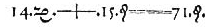Home Practice
For learners and parents For teachers and schools
Textbooks
Full catalogue
Pricing SupportLog in

We think you are located in United States. Is this correct?

# Test yourself now

High marks in science are the key to your success and future plans. Test yourself and learn more on Siyavula Practice.

# Chapter 4: Equations and inequalities

## 4.1 Introduction (EMA33)

• This chapter covers linear, quadratic and simultaneous linear equations as well as word problems, literal equations and linear inequalities.
• Linear equations were covered in earlier grades and are revised here.
• Word problems can include any of linear, quadratic and simultaneous equations.
• For linear inequalities learners must know interval notation and be able to represent the solution graphically.

Equations are widely used to describe the world around us. In science equations are used to describe everything from how a ball rolls down a slope to how the planets move around the sun.

In this chapter we will explore different types of equations as well as looking at how these can be used to solve problems in the real world. We will also look at linear inequalities.The first use of an “equals” sign from The Whetstone of Witte by Robert Recorde $$1557$$. This equation represents $$14x + 15 = 71$$. Recorde is also responsible for introducing the pre-existing “plus” sign (+) to the English-speaking world.